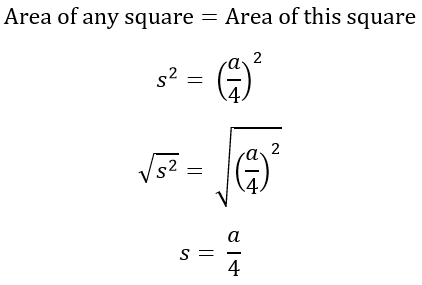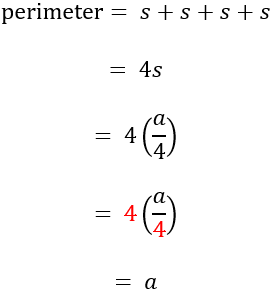Search
• nokwareknight

# SAT Math: Using Surface Area of Cube to Find Perimeter of a Face

Updated: Mar 9

This question comes from Practice Test 5, Section 4 Calculator, #26.Starting off, we know we are going to use the surface area of a cube to get the perimeter of one face of a cube.

A cube is a 3D figure made of six squares. Something like below (you do not need to draw it to solve the problem; it just may help to visualize).Each side is a square of equal size. So the total surface area can be divided into six equal parts to find the surface area for one side of the cube.Compare this to the general equation for the area of a square, using s to represent one side of the square.To find the perimeter of a square, we need to add up the lengths of its 4 sides. Since the sides of a square are all equal, multiplying by 4 will work as well.-Nokware Knight